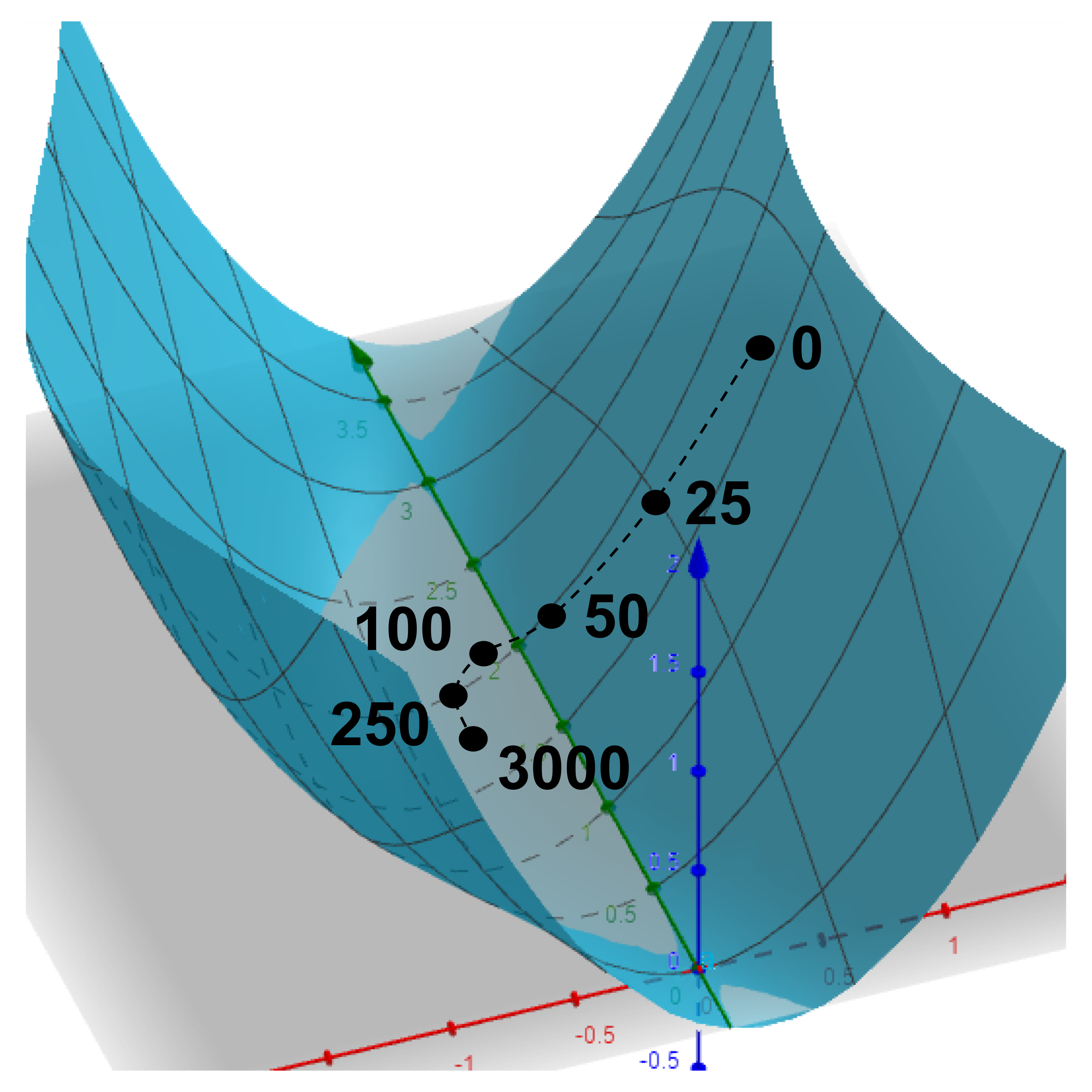Just like single-variable gradient descent, except that we replace the derivative with the gradient vector.

This post is part of a series.

Multivariable gradient descent is similar to single-variable gradient descent, except that we replace the derivative $f’(x)$ with the gradient $\nabla f(\vec x)$ as follows:

\begin{align*} \vec x_{n+1} = \vec x_n - \alpha \nabla f(\vec x_n) \end{align*}

Here, $\vec x_n$ denotes the vector of $n$th guesses for all the variables. For example, if $f$ is a function of $2$ input variables $x,y,$ then we denote

\begin{align*} \vec x_n = \left< x_n,y_n \right> \end{align*}

and the update rule can be expressed as follows:

\begin{align*} \left< x_{n+1}, y_{n+1} \right> &= \left< x_n, y_n \right> - \alpha \nabla f(x_n, y_n) \\[5pt] &= \left< x_n, y_n \right> - \alpha \left. \left< \dfrac{\partial f}{\partial x}, \dfrac{\partial f}{\partial y} \right> \right|_{(x_n, y_n)} \end{align*}

## Worked Example

To illustrate, let’s use gradient descent to minimize the following function:

\begin{align*} f(x,y) = x \sin y + x^2 \end{align*}

First, we work out the gradient:

\begin{align*} \nabla f(x,y) &= \left< \dfrac{\partial f}{\partial x}, \dfrac{\partial f}{\partial y} \right> \\[5pt] &= \left< \dfrac{\partial}{\partial x} \left( x \sin y + x^2 \right), \dfrac{\partial}{\partial y} \left( x \sin y + x^2 \right) \right> \\[5pt] &= \left< \sin y + 2x, x \cos y \right> \end{align*}

If we start with the initial guess $x=1, y=2$ (which we denote as $\left< x,y \right>_0 = \left< 1,2 \right>$) and use a learning rate of $\alpha = 0.01,$ then our next guess is

\begin{align*} \left< x_1,y_1 \right> &= \left< x_0,y_0 \right> - \alpha \nabla f(x_0,y_0) \\[5pt] &= \left< 1,2 \right> - \left. (0.01) \left< \sin y + 2x, x \cos y \right> \right|_{(1,2)} \\[5pt] &= \left< 1,2 \right> - (0.01) \left< \sin 2 + 2, \cos 2 \right> \\[5pt] &\approx \left< 0.970907, 2.004161 \right>. \end{align*}

We will carry out the rest of the iterations using a computer program. You should do this too and verify that you get the same results as shown in the table below. In the table, we will round to $6$ decimal places (but we do not actually round in our computer program).

\begin{align*} \begin{matrix} n & \left< x_n, y_n \right> & \nabla f(x_n,y_n) \\ \hline 0 & \left< 1, 2 \right> & \left< 2.909297, -0.416147 \right> \\ 1 & \left< 0.970907, 2.004161 \right> & \left< 2.849372, -0.40771 \right> \\ 2 & \left< 0.942413, 2.008239 \right> & \left< 2.790665, -0.399229 \right> \\ 3 & \left< 0.914507, 2.012231 \right> & \left< 2.733153, -0.390711 \right> \\ \vdots & \vdots & \vdots & \\ 25 & \left< 0.427158, 2.078619 \right> & \left< 1.728121, -0.207717 \right> \\ 50 & \left< 0.086462, 2.109688 \right> & \left< 1.031202, -0.044371 \right> \\ 100 & \left< -0.242655, 2.084195 \right> & \left< 0.385770, 0.119178 \right> \\ 250 & \left< -0.457667, 1.862063 \right> & \left< 0.042547, 0.131426 \right> \\ 500 & \left< -0.496295, 1.657935 \right> & \left< 0.003616, 0.043192 \right> \\ 1000 & \left< -0.499975, 1.577936 \right> & \left< 0.000025, 0.003569 \right> \\ 2000 & \left< -0.500000, 1.570844 \right> & \left< 0.000000, 0.000024 \right> \\ 3000 & \left< -0.500000, 1.570797 \right> & \left< 0.000000, 0.000000 \right> \\ \end{matrix} \end{align*}

If we plot graph of the surface and some of our intermediate guesses, we can see that our guesses do indeed take us down the valley into a minimum:## Sanity Check

When we run gradient descent on functions with more than two input variables, it becomes difficult to visualize the graph of the function. However, we can still verify that we’re moving in the correct direction by evaluating the function at each guess and making sure the function values are decreasing.

To illustrate, let’s add another column $f(x_n,y_n)$ on the right side of the table above. We can see that the function values in this column are decreasing, and this tells us that we are successfully minimizing our function $f.$

\begin{align*} \begin{matrix} n & \left< x_n, y_n \right> & \nabla f(x_n,y_n) & \big\vert & f(x_n,y_n) \\ \hline 0 & \left< 1, 2 \right> & \left< 2.909297, -0.416147 \right> & \big\vert & 1.909297 \\ 1 & \left< 0.970907, 2.004161 \right> & \left< 2.849372, -0.40771 \right> & \big\vert & 1.823815 \\ 2 & \left< 0.942413, 2.008239 \right> & \left< 2.790665, -0.399229 \right> & \big\vert & 1.741817 \\ 3 & \left< 0.914507, 2.012231 \right> & \left< 2.733153, -0.390711 \right> & \big\vert & 1.663164 \\ \vdots & \vdots & \vdots & \Bigg\vert & \vdots \\ 25 & \left< 0.427158, 2.078619 \right> & \left< 1.728121, -0.207717 \right> & \big\vert & 0.555717 \\ 50 & \left< 0.086462, 2.109688 \right> & \left< 1.031202, -0.044371 \right> & \big\vert & 0.081684 \\ 100 & \left< -0.242655, 2.084195 \right> & \left< 0.385770, 0.119178 \right> & \big\vert & -0.152491 \\ 250 & \left< -0.457667, 1.862063 \right> & \left< 0.042547, 0.131426 \right> & \big\vert & -0.228931 \\ 500 & \left< -0.496295, 1.657935 \right> & \left< 0.003616, 0.043192 \right> & \big\vert & -0.248103 \\ 1000 & \left< -0.499975, 1.577936 \right> & \left< 0.000025, 0.003569 \right> & \big\vert & -0.249987 \\ 2000 & \left< -0.500000, 1.570844 \right> & \left< 0.000000, 0.000024 \right> & \big\vert & -0.250000 \\ 3000 & \left< -0.500000, 1.570797 \right> & \left< 0.000000, 0.000000 \right> & \big\vert & -0.250000 \\ \end{matrix} \end{align*}

## Exercises

Use gradient descent to minimize the following functions. Use several different initial guesses, and then plug your final guesses back into the function to determine which final guess is the best (i.e. gives you the lowest function value). Be sure to evaluate the function at each guess and verify that the function values are decreasing.

1. $f(x,y) = (x-1)^2 + 3y^2$
2. $f(x,y) = y^2 + y \cos x$
3. $f(x,y,z) = (x-1)^2 + 3(y-2)^2 + 4(z+1)^2$
4. $f(x,y,z) = x^2 + 3y^2 + 4z^2 + \cos(xyz)$

This post is part of a series.

Tags: